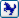Join the forum, it's quick and easy

Would you like to react to this message? Create an account in a few clicks or log in to continue.

# [討論]分享即時顯示聚合線長度LISP

+5
Rocky33321
mayaguy
regina
Tiger&蘋果爸
devinchou
9 posters##[討論]分享即時顯示聚合線長度LISP

`　;　[AutoCAD EXTEND] SHOWN IN LENGTH OF PLINE.　;　[AutoCAD "指令"功能延伸] 即時顯示聚合線長度。　;　支援軟體版本("AutoCAD")　;　設計者：Devin,Chou　;　版權所有，歡迎使用　;　Rev.1(2016/11/27-12/03)(defun C:pls (/ pl_o pl_d pl_10 pl_70                d_p d_lh x_p x_lh xy_lt                pt1 pt2 pl_dt                t_lay )(setvar "cmdecho" 1 )(setq t_lay    (tblsearch "LAYER" "TEMP" ))(if (= t_lay nil )    (progn    (setq t_lay "TEMP" )    (entmake    (list    '(0 . "LAYER" )    '(100 . "AcDbSymbolTableRecord" )    '(100 . "AcDbLayerTableRecord" )    '(62 . 7 )    '(70 . 0 )    '(6 . "Continuous" )     (cons 2 t_lay )))) ; progn.end    (setq t_lay "TEMP" )) ; if.end(setq xy_lt '() d_p 0 x_p 0 )(command "PLINE"    (while (> (getvar 'cmdactive ) 0 )    (command pause ))) ; while.end,pline.end(setq pl_o    (entlast ))(setq pl_d    (entget pl_o ))(setq pl_70    (cdr    (assoc 70 pl_d )))(setq d_lh    (length pl_d ))(while (< d_p d_lh )(setq pl_10    (nth d_p pl_d ))(if (= (car pl_10 ) 10 )    (setq xy_lt    (cons pl_10 xy_lt ))) ; if.end(setq d_p    (1+ d_p ))) ; while.end(setq xy_lt    (reverse xy_lt ))(setq x_lh    (length xy_lt ))(if (= pl_70 0 )    (progn    (while (< x_p (- x_lh 1 ))    (setq pt1    (cdr    (nth x_p xy_lt )))    (setq pt2    (cdr    (nth (1+ x_p ) xy_lt )))    (setq x_p    (1+ x_p ))    (setq pl_dt    (rtos (distance pt1 pt2 ) 2 0 ))    (entmake_text )))) ; while.end,progn.end,if.end(if (= pl_70 1 )    (progn    (while (< x_p x_lh )    (setq pt1    (cdr    (nth x_p xy_lt )))    (if (< (1+ x_p ) x_lh )    (setq pt2    (cdr    (nth (1+ x_p ) xy_lt )))    (setq pt2    (cdr    (nth 0 xy_lt )))) ; if.end    (setq x_p    (1+ x_p ))    (setq pl_dt    (rtos (distance pt1 pt2 ) 2 0 ))    (entmake_text )))) ; while.end,progn.end,if.end(prin1)) ; pls.end,主程式結束;－－－－－－－－－－主副程式分隔線－－－－－－－－－－;(defun entmake_text ()(entmake    (list    '(0 . "TEXT" )     (cons 1 pl_dt )     (cons 10 pt1 )     (cons 40 20 )     (cons 8 t_lay )))(prin1)) ; entmake_text.end,副程式結束(princ "\nC:pls")(prin1)`devinchou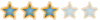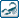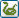zx3613133 likes this post

##回復： [討論]分享即時顯示聚合線長度LISPTiger&蘋果爸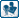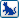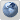##回復： [討論]分享即時顯示聚合線長度LISPregina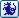##回復： [討論]分享即時顯示聚合線長度LISPdevinchou##回復： [討論]分享即時顯示聚合線長度LISPmayaguy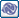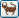##回復： [討論]分享即時顯示聚合線長度LISP

mayaguy 寫到:感謝分享！這很適合用在建築圖說的步行距離，只要把文字位置改到線段中央就完美了，我可以自己修改使用嗎？devinchou##回復： [討論]分享即時顯示聚合線長度LISP

devinchou 寫到:
mayaguy 寫到:感謝分享！這很適合用在建築圖說的步行距離，只要把文字位置改到線段中央就完美了，我可以自己修改使用嗎？mayaguy##回復： [討論]分享即時顯示聚合線長度LISPRocky33321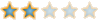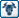##回復： [討論]分享即時顯示聚合線長度LISPlinxyz168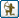##直線+三點畫弧, 我自己寫過的程式我都忘記了, 哈 ... 參考一下. 3Q : )shackle_2005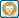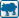##回復： [討論]分享即時顯示聚合線長度LISP感謝分享box888##回復： [討論]分享即時顯示聚合線長度LISPrickyyang